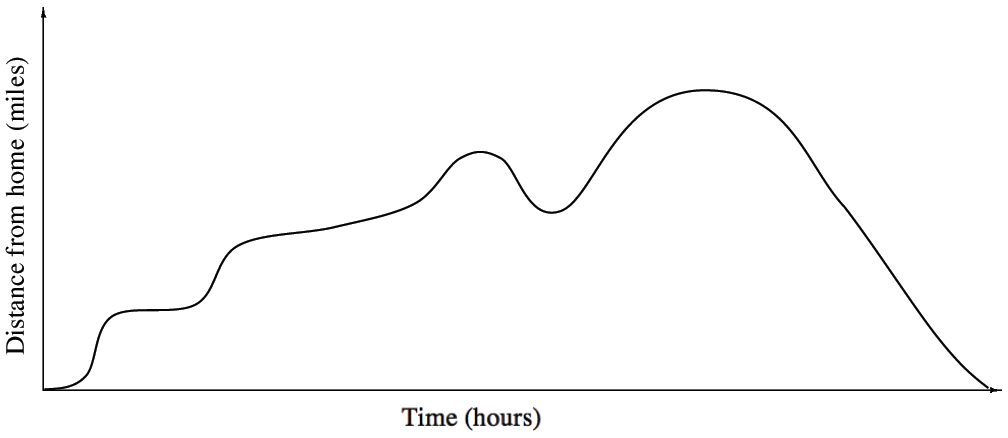### Home > APCALC > Chapter 3 > Lesson 3.2.1 > Problem3-50

3-50.

WHAT A DAY!

Below is a graph of the distance David traveled away from his home on a trip to the mountains. Place the events listed at right in the proper order based on details from the graph.

Trace the graph below and identify the parts that correspond to each event during David’s trip.

Then answer parts (a) through (d) below.

The four places where events take place are the places where the slope changes:
1) The spot where the graph flattens out momentarily.
2) The long horizontal section of the graph.
3) Where the slope is negative for a short time.
4) Where the slope is negative for a long time.

 EVENTSDavid has to drive back to pick up his credit card that he forgot at the restaurant.David’s car breaks down and he is towed back to a repair shop near his house.David stops for gas and gets a quick bite to eat.David gets pulled over and receives a speeding ticket. He then continues his trip at a slower rate.1. What are the units for the slope of the curve?

$\text{Slope} = \frac{\text Rise}{\text Run}=\frac{\Delta x}{\Delta y}=\frac{\text{Units on the } x \text{ axis}}{\text{Units on the } y \text { axis}}=?$

2. What is significant about the slope of the curve when David is stopped?

moving forward → positive slope
moving backward → negative slope
stopping → __________

3. How does the slope of the curve tell you when David is speeding?

If velocity is slope of the distance graph... so speeding would mean steep slope. Be mindful that speeding could mean steep-and-positive or steep-and-negative.

4. Interpret the graph where the slope is negative. What is David doing then?

Refer to the hint in part (b).